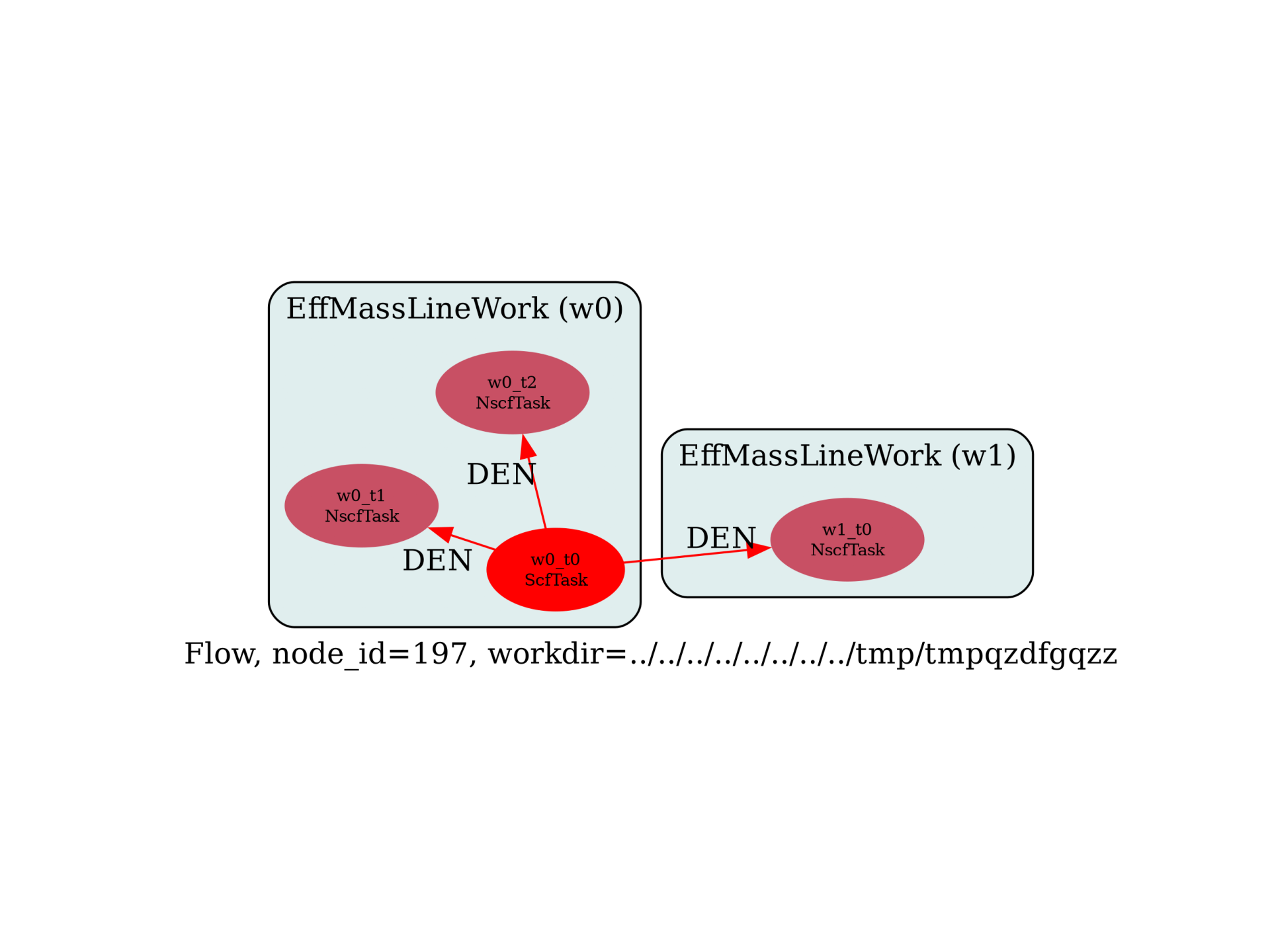# Effective masses with finite difference¶

Flow to compute effective masses with finite difference method. Derivatives are computed along lines in k-space.

import sys
import os
import abipy.data as abidata
import abipy.abilab as abilab
import abipy.flowtk as flowtk

def make_scf_input(nspinor=1, usepaw=0):
"""Returns GS input file for Silicon"""
if nspinor == 1:
pseudos = abidata.pseudos("14si.pspnc") if usepaw == 0 else abidata.pseudos("Si.GGA_PBE-JTH-paw.xml")
else:
pseudos = abidata.pseudos("Si_r.psp8") if usepaw == 0 else abidata.pseudos("Si.GGA_PBE-JTH-paw.xml")

structure = dict(
ntypat=1,
natom=2,
typat=[1, 1],
znucl=14,
#acell=3 * [10.26310667319252], # https://docs.abinit.org/tests/v7/Input/t82.in
acell=3 * [10.2073557], # 5.4015 Ang
rprim=[[0.0,  0.5,  0.5],
[0.5,  0.0,  0.5],
[0.5,  0.5,  0.0]],
xred=[ [0.0 , 0.0 , 0.0],
[0.25, 0.25, 0.25]],
)

# Build input
scf_input = abilab.AbinitInput(structure=structure, pseudos=pseudos)

# Set variables
scf_input.set_vars(
ecut=12,
nband=8 if nspinor == 1 else 16,
nspinor=nspinor,
tolvrs=1e-8,
)

if scf_input.ispaw:
scf_input.set_vars(pawecutdg=2 * scf_input["ecut"])

scf_input.set_kmesh(ngkpt=[8, 8, 8], shiftk=[0, 0, 0])

return scf_input

def build_flow(options):
# Set working directory (default is the name of the script with '.py' removed and "run_" replaced by "flow_")
if not options.workdir:
options.workdir = os.path.basename(sys.argv).replace(".py", "").replace("run_", "flow_")

# Get the SCF input (here NC with SOC)
scf_input = make_scf_input(nspinor=2, usepaw=0)

# Build the flow with different steps.
from abipy.flowtk.effmass_works import EffMassLineWork
flow = flowtk.Flow(workdir=options.workdir, manager=options.manager)

# Multiple calculations with different step for finite difference.
for i, step in enumerate((0.01, 0.005)):
if i == 0: den_node = None
work = EffMassLineWork.from_scf_input(scf_input, k0_list=(0, 0, 0),
step=step, npts=10,
red_dirs=[[1, 0, 0], [1, 1, 0]],
cart_dirs=[[1, 0, 0], [1, 1, 1], [1, 1, 0]],
den_node=den_node)
# Will start from the DEN file produced in the first iteration.
if i == 0: den_node = work
flow.register_work(work)

return flow

# This block generates the thumbnails in the Abipy gallery.
# You can safely REMOVE this part if you are using this script for production runs.
__name__ = None
import tempfile
options = flowtk.build_flow_main_parser().parse_args(["-w", tempfile.mkdtemp()])
build_flow(options).graphviz_imshow()

@flowtk.flow_main
def main(options):
"""
This is our main function that will be invoked by the script.
flow_main is a decorator implementing the command line interface.
Command line args are stored in options.
"""
return build_flow(options)

if __name__ == "__main__":
sys.exit(main())Out:

/Users/gmatteo/git_repos/pymatgen/pymatgen/util/plotting.py:550: UserWarning: Matplotlib is currently using agg, which is a non-GUI backend, so cannot show the figure.
plt.show()


Run the script with:

run_effmass_finitediff -s

Total running time of the script: ( 0 minutes 0.514 seconds)

Gallery generated by Sphinx-Gallery